# FORECAST Function

Calculates a future value using existing values

## What is the FORECAST Function?

The FORECAST Function is categorized under Excel Statistical functions. It will calculate or predict a future value using existing values.

In financial modeling, the FORECAST function can be useful in calculating the statistical value of a forecast made. For example, if we know the past earnings and expenses, we can forecast the future amounts using the function.

### Formula

=FORECAST(x, known_y’s, known_x’s)

The FORECAST function uses the following arguments:

1. X (required argument) – This is a numeric x-value for which we want to forecast a new y-value.
2. Known_y’s (required argument) – The dependent array or range of data.
3. Known_x’s (required argument) – This is the independent array or range of data that is known to us.

### How to use the FORECAST Function in Excel?

As a worksheet function, FORECAST can be entered as part of a formula in a cell of a worksheet. To understand the uses of the function, let’s consider an example:

#### Example

Suppose we are given earnings data, which are the known x’s, and expenses, which are the known y’s. We can use the FORECAST function to predict an additional point along the straight line of best fit through a set of known x- and y-values. Using the data below: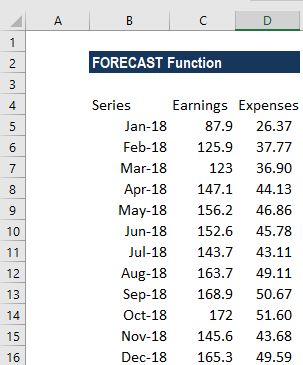Using earnings data for January 2019, we can predict the expenses for the same month using the FORECAST function.

The formula to use is: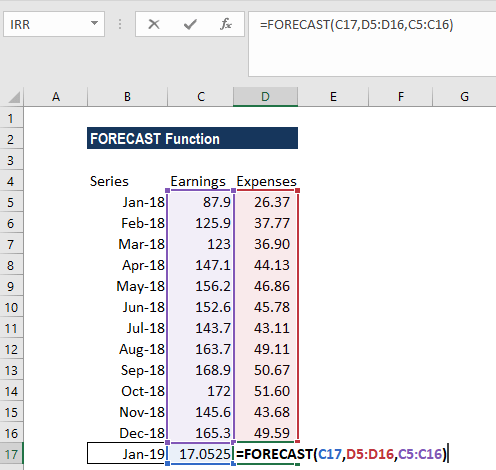We get the results below: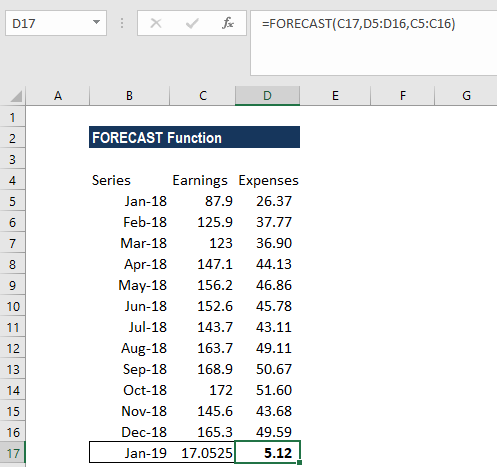The FORECAST function will calculate a new y-value using the simple straight-line equation: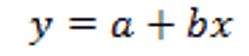Where: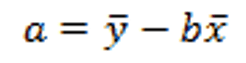and: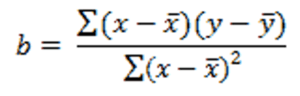The values of x and y are the sample means (the averages) of the known x- and the known y-values.

### A few notes about the function:

1. The length of the known_x’s array should be the same length as the known_y’s, and the variance of the known_x’s must not be zero.
2. #N/A! error – Occurs if:
1. The supplied values known_x’s and the supplied known_y’s arrays have different lengths.
2. One or both of the known_x’s or the known_y’s arrays are empty.
1. #DIV/0! error – Occurs if the variance of the supplied known_x’s is equal to zero.
2. #VALUE! error – Occurs if the given future value of x is non-numeric.